# How to create a spell check enabled MySQL query by leveraging SOUNDEX and Levenshtein Distance algorithms

Recently I came across a situation where I needed to perform the MySQL search in such way, that it would account for typos in user search queries. For example, if the database of words in MySQL contained only the word “assistance” and the user typed the misspelled word “asistence”, I had to be able to return the correct word “assistance” as a closest possible suggestion from the database. It seemed like a trivial task at first, but it took me quite a while to figure the SQL query that had a good balance between performance and overall quality of results.

The solutions I found on the internet were frequently suggesting the SOUNDEX phonetic algorithm built into MySQL.  SOUNDEX was originally developed by Robert C. Russell and Margaret King in the early 1900’s, with the goal to match the words despite minor differences in spelling.

I’ve fiddled with SOUNDEX for a while and considering the results of my testing, I concluded that SOUNDEX provided suggestions that were completely unreliable. Just take a look at the following experiment when running a SOUNDEX based query on the database of almost half a million English words using a misspelled word: “asistence”

`SELECT * FROM en_english479k WHERE SOUNDEX(word) like SOUNDEX("asistence") limit 10`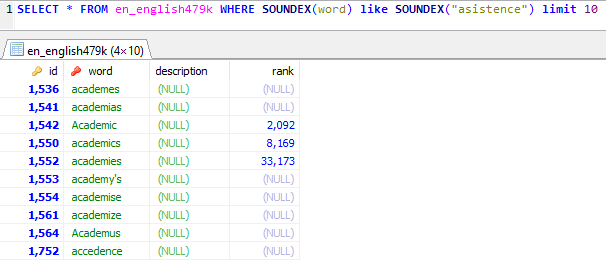As you can see, SOUNDEX is completely off, even if I use the sorting by English rank which I added into the database to assist with finding the most common English words: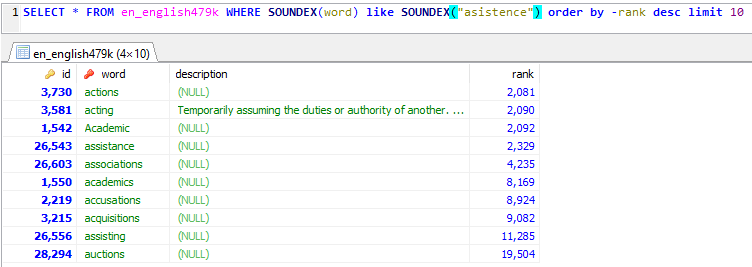Considering the results, I’ve decided to abandon SOUNDEX and see if there are other options out there, which brought me to Levenshtein distance (LD) algorithm.

Levenshtein distance (LD) is a degree of the similarity between two strings. The distance is the number of deletions, insertions, or substitutions required to transform source string into the target string. The Levenshtein distance algorithm has been used in: Spell checking, Speech recognition, DNA analysis and Plagiarism detection.

For example, if the source string is (s) and the target string is (t), then:

• If s is “assistance” and t is “assistance”, then LD(s,t) = 0, because no transformations are needed. The strings are already identical.
• If s is “assistance” and t is “asistence”, then LD(s,t) = 2, because two substitutions or changes are required to transform s into t.

NOTE: You can use https://planetcalc.com/1721/ to calculate Levenshtein distance between two strings if you want to play with it.

Anyhow, this seemed like a brilliant solution, however, MySQL doesn’t include a native Levenshtein Distance function. That said, I found the MySQL function on Github, which I’ve added to my database. This is it:

```-- Levenshtein function
-- Source: https://openquery.com.au/blog/levenshtein-mysql-stored-function
-- Levenshtein reference: http://en.wikipedia.org/wiki/Levenshtein_distance

-- Arjen note: because the levenshtein value is encoded in a byte array, distance cannot exceed 255;
-- thus the maximum string length this implementation can handle is also limited to 255 characters.

DELIMITER \$\$
DROP FUNCTION IF EXISTS LEVENSHTEIN \$\$
CREATE FUNCTION LEVENSHTEIN(s1 VARCHAR(255) CHARACTER SET utf8, s2 VARCHAR(255) CHARACTER SET utf8)
RETURNS INT
DETERMINISTIC
BEGIN
DECLARE s1_len, s2_len, i, j, c, c_temp, cost INT;
DECLARE s1_char CHAR CHARACTER SET utf8;
-- max strlen=255 for this function
DECLARE cv0, cv1 VARBINARY(256);

SET s1_len = CHAR_LENGTH(s1),
s2_len = CHAR_LENGTH(s2),
cv1 = 0x00,
j = 1,
i = 1,
c = 0;

IF (s1 = s2) THEN
RETURN (0);
ELSEIF (s1_len = 0) THEN
RETURN (s2_len);
ELSEIF (s2_len = 0) THEN
RETURN (s1_len);
END IF;

WHILE (j <= s2_len) DO
SET cv1 = CONCAT(cv1, CHAR(j)),
j = j + 1;
END WHILE;

WHILE (i <= s1_len) DO
SET s1_char = SUBSTRING(s1, i, 1),
c = i,
cv0 = CHAR(i),
j = 1;

WHILE (j <= s2_len) DO
SET c = c + 1,
cost = IF(s1_char = SUBSTRING(s2, j, 1), 0, 1);

SET c_temp = ORD(SUBSTRING(cv1, j, 1)) + cost;
IF (c > c_temp) THEN
SET c = c_temp;
END IF;

SET c_temp = ORD(SUBSTRING(cv1, j+1, 1)) + 1;
IF (c > c_temp) THEN
SET c = c_temp;
END IF;

SET cv0 = CONCAT(cv0, CHAR(c)),
j = j + 1;
END WHILE;

SET cv1 = cv0,
i = i + 1;
END WHILE;

RETURN (c);
END \$\$

DELIMITER ;```

Once I added Levenshtein function into my database, I decided to try it out, by running a query like this, also leveraging rank and Levenshtein ordering to get the results just right:

`SELECT * FROM en_english479k WHERE levenshtein(word, "asistence")<=2 limit 3`

This worked great: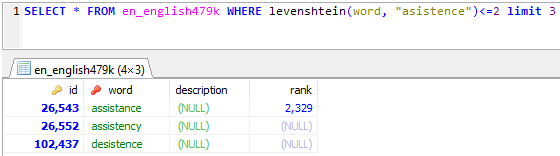Except it was entirely unusable because even though the query returned a correct result, it was incredibly slow. Look at the time required to find the result:

`SELECT * FROM en_english479k WHERE levenshtein(word, "asistence")<=2 limit 1;`

RESULT: /* Affected rows: 0 Found rows: 1 Warnings: 0 Duration for 1 query: 17.235 sec. */

`SELECT * FROM en_english479k WHERE levenshtein(word, "asistence")<=2 limit 3;`

RESULT: /* Affected rows: 0 Found rows: 3 Warnings: 0 Duration for 1 query: 00:01:07 minute*/

The time required to find more results is growing exponentially with the number of required results.

This took me back to the drawing board. The final solution used a combination of SOUNDEX and LEVENSHTEIN algorithms to get the correct result and get them fast, leveraging not only

```SELECT *
FROM en_english479k
WHERE SOUNDEX(word) LIKE SOUNDEX("asistence") AND levenshtein(word, "asistence")<=2
ORDER BY -rank DESC, -levenshtein(word, "asistence") DESC
LIMIT 1```

The result was found almost immediately, within 0.29 seconds on my 8GB MySQL test box using the Limit 1: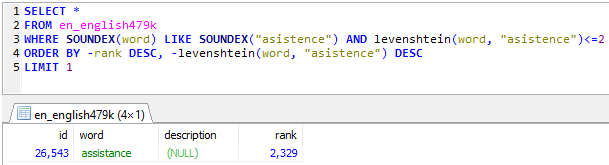Removing the limit completely to search through an entire database of half a million English words took only 0.31 seconds: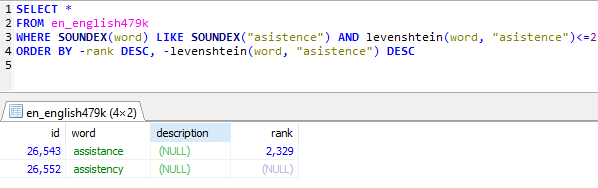So, the conclusion is to use both methods. The first SOUNDEX gets you the words that sound similar and then LEVENSHTEIN does a good job of filtering these results down to a correct word that most closely matches the typo or misspelling in the user’s entry.

In my testing, the results I am getting out of this query are on par with Bing Spell Search API v7 without paying a cent for such a service and without incurring the latency cost associated with running a spell checking service from the cloud.

Note on Misspelled vs Mistyped

The above solution works very well for searches where the misspelled word sounds like the original word, which is the case most of the time. However, if the misspelled word does not sound like the original word, then the logic of using SOUNDEX breaks. So for example, if we’re searching for ‘assistance’ by looking up ‘qssistance’ it no longer sounds like the original word and SOUNDEX becomes mostly useless. Even though not many people actually do that type of mistake, it’s possible to mistype the word this way, as Q is very close to A on the physical keyboard. To fix such an issue, one could still rely purely on Levenshtein which would get the job done, albeit it’d be a lot slower. In order to speed it up, you’d need to create a separate column in which you’d start capturing the misspelled combinations for each word in your database and always look up existing ‘captured’ combinations prior to an actual search, to make repeating future searches a bit faster.